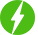Optimization Problem
• Show all
• Hottest
• Popular
0

## Power System Optimization in Pyomo (Part 1)

Power System Optimization in Pyomo (Part 1), Learn ED, DED, Battery model, Wind, Sensitivity Analsysis, and Visualization modeling in Pyomo. Course ...

Expand
5

## Pyomo Bootcamp: Python Optimization from Beginner to Advance

Pyomo Bootcamp: Python Optimization from Beginner to Advance, Guide for building optimization problem (operation research) in Pyomo Jupyter and solve it using ...

Expand
2

## Complete guide to Modern Mathematical Optimization in GAMS

Complete guide to Modern Mathematical Optimization in GAMS, From Zero to Hero. Course Description The developed course is suitable for you even if ...

Expand
1

## Solving the Optimal Power Flow Optimization problem (DC/AC)

Solving the Optimal Power Flow Optimization problem (DC/AC), GAMS and Pyomo. Course Description Optimal power flow (OPF) is a mathematical ...

Expand
1

## Basics of Optimization in Engineering

Basics of Optimization in Engineering, Optimization problems definition and solution . Optimal design in different engineering systems. Course Description ...

Expand
2

## Mathematical Optimization with GAMS and Pyomo (Python)

Mathematical Optimization with GAMS and Pyomo (Python) Learn how to mathematically formulate 16 business problems and find their optimal solutions with GAMS ...

ExpandRegister New Account
• Total (0)
0